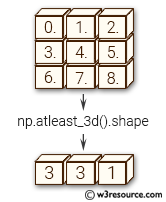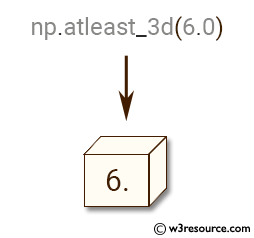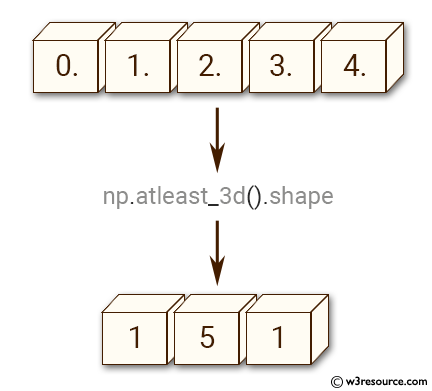# NumPy Array manipulation: atleast_3d() function

## numpy.atleast_3d() function

The numpy.atleast_3d() function is used to view given inputs as arrays with at least three dimensions.

Syntax:

`numpy.atleast_3d(*arys)`Version: 1.15.0

Parameter:

Name Description Required /
Optional
arys1, arys2, . . . One or more array-like sequences. Non-array inputs are converted
to arrays. Arrays that already have three or more dimensions are preserved.
Required

Return value:

res1, res2, . . . [ndarray]

Example-1: numpy.atleast_3d()

``````>>> import numpy as np
>>> np.atleast_3d(6.0)
array([[[ 6.]]])
``````

Pictorial Presentation:Example-2: numpy.atleast_3d()

``````>>> import numpy as np
>>> y = np.arange(5.0)
>>> np.atleast_3d(y).shape
(1, 5, 1)
``````

Pictorial Presentation:Example-3: numpy.atleast_3d()

``````>>> import numpy as np
>>> y = np.arange(9.0).reshape(3,3)
>>> np.atleast_3d(y).shape
(3, 3, 1)
>>> np.atleast_3d(y).base is y.base
True
>>> for arr in np.atleast_3d([1,2],[[1,2]],[[[1,2]]]):
...   print(arr, arr.shape)
...
[[
]] (1, 2, 1)
[[
]] (1, 2, 1)
[[[1 2]]] (1, 1, 2)
``````

Python - NumPy Code Editor:

Previous: arleast_2d()
Next: broadcast

﻿